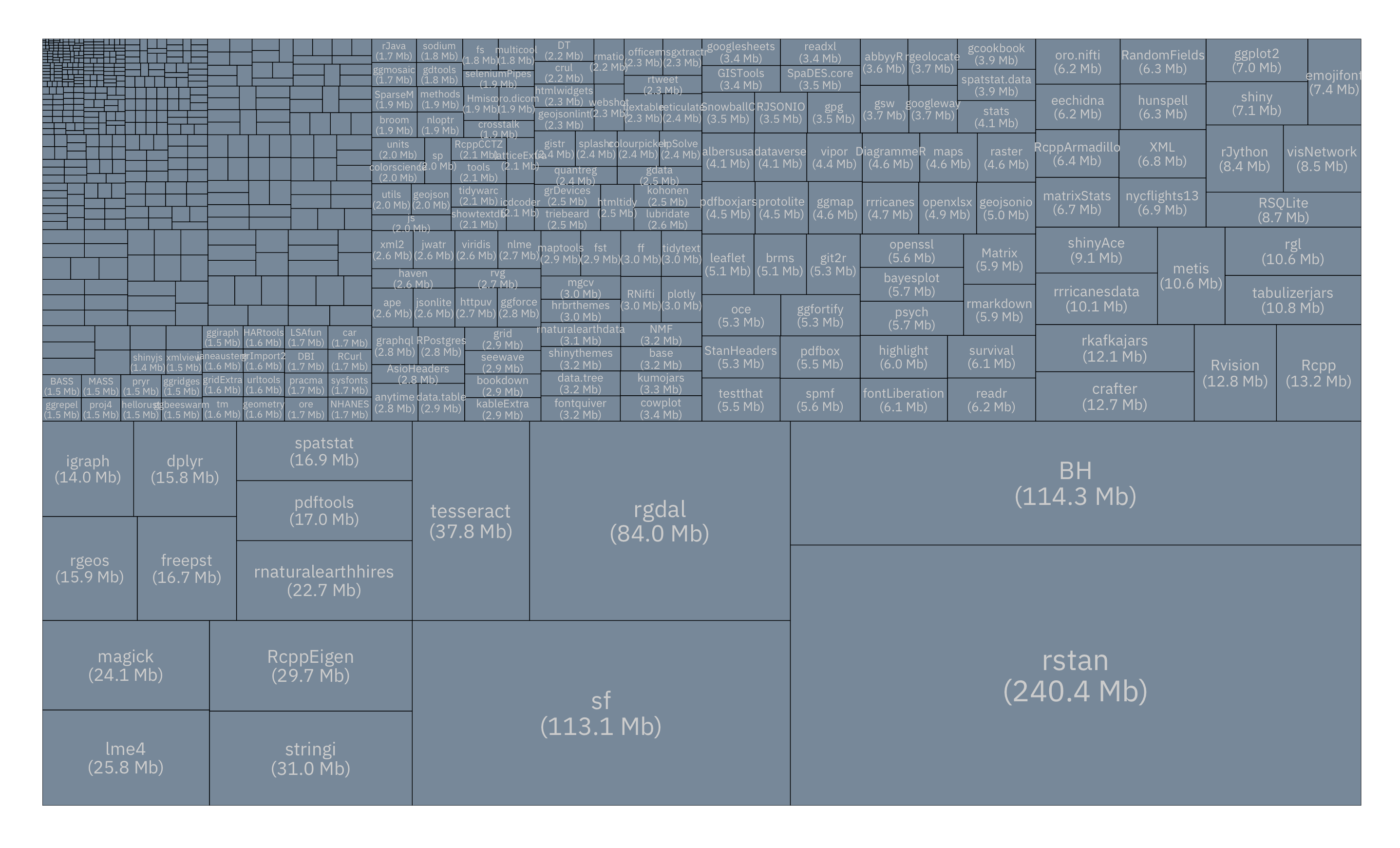# Compute/Visualize Drive Space Consumption of Your Installed R Packages

The `fs` package makes it super quick and easy to find out just how much “package hoarding” you’ve been doing:

``````library(fs)
library(ggalt) # devtools::install_github("hrbrmstr/ggalt")
library(igraph)
library(ggraph) # devtools::install_github("thomasp85/ggraph")
library(hrbrthemes) # devtools::install_github("hrbrmstr/hrbrthemes")
library(tidyverse)

installed.packages() %>%
as_data_frame() %>%
mutate(pkg_dir = sprintf("%s/%s", LibPath, Package)) %>%
select(pkg_dir) %>%
mutate(pkg_dir_size = map_dbl(pkg_dir, ~{
fs::dir_info(.x, all=TRUE, recursive=TRUE) %>%
summarise(tot_dir_size = sum(size)) %>%
pull(tot_dir_size)
})) %>%
summarise(
total_size_of_all_installed_packages=ggalt::Gb(sum(pkg_dir_size))
) %>%
unlist()
## total_size_of_all_installed_packages
##                             "1.6 Gb"``````

While you can modify the above and peruse the list of packages/directories in tabular format or programmatically, you can also do a bit more work to get a visual overview of package size (click/tap the image for a larger view):

``````installed.packages() %>%
as_data_frame() %>%
mutate(pkg_dir = sprintf("%s/%s", LibPath, Package)) %>%
mutate(dir_info = map(pkg_dir, fs::dir_info, all=TRUE, recursive=TRUE)) %>%
mutate(dir_size = map_dbl(dir_info, ~sum(.x\$size))) -> xdf

select(xdf, Package, dir_size) %>%
mutate(grp = "ROOT") %>%
add_row(grp = "ROOT", Package="ROOT", dir_size=0) %>%
select(grp, Package, dir_size) %>%
arrange(desc(dir_size)) -> gdf

select(gdf, -grp) %>%
mutate(lab = sprintf("%s\n(%s)", Package, ggalt::Mb(dir_size))) %>%
mutate(lab = ifelse(dir_size > 1500000, lab, "")) -> vdf

g <- graph_from_data_frame(gdf, vertices=vdf)

ggraph(g, "treemap", weight=dir_size) +
geom_node_tile(fill="lightslategray", size=0.25) +
geom_text(
aes(x, y, label=lab, size=dir_size),
color="#cccccc", family=font_ps, lineheight=0.875
) +
scale_x_reverse(expand=c(0,0)) +
scale_y_continuous(expand=c(0,0)) +
scale_size_continuous(trans="sqrt", range = c(0.5, 8)) +
ggraph::theme_graph(base_family = font_ps) +
theme(legend.position="none")``````### Challenge

Do some wrangling with the above data and turn it into a package “disk explorer” with @timelyportfolio’s `d3treeR`? package.Amazon Author Page

## 6 Comments →Compute/Visualize Drive Space Consumption of Your Installed R Packages

1.jixing

object ‘dir_size’ not found for ” ggraph(g, “treemap”, weight=dir_size) “

1.hrbrmstr

The post indicates that `ggraph` was the github version. Did you install it from github?

1.aurelien

I guess the typo in `devtools::install_github("thomasp85/igraph")` might have responsible for the confusion. Easy enough to correct `igraph` in `ggraph` though. Thanks for sharing this Robert!

2.Craig Lewis

The source code references loading igraph from github (via devtools::install_github(“thomasp85/igraph”) this yielded a 404 error for me:
from URL https://api.github.com/repos/thomasp85/igraph/zipball/master

I then tried to install ggraph from github – this installed okay but the dir_size error remained.

Nice article – would like to get this code to run!

1.hrbrmstr

Try `devtools::install_github("thomasp85/ggraph")`. The original post had `igraph` vs `ggraph` and that was was a typo.

I’ve run it (using that dev version) in a vanilla R session on macOS and Linux and iut worked.

3.chinsoon12

+nice. The SpaceSniffer program does something similar

This site uses Akismet to reduce spam. Learn how your comment data is processed.